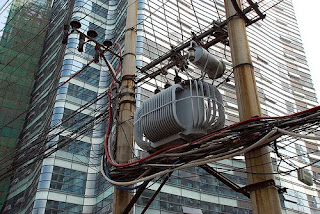# All Day Efficiency of a Distribution Transformer

The commercial Efficiency of a transformer is given by the ratio of output power to input power

Efficiency = output power in watts/input power in watts

The losses in the transformer can be classified into copper losses and iron losses. The copper losses (hysteresis and Eddy Current Losses) are independent of the load. The iron losses though are dependent on the load.In the case of the distribution transformers, the load is continually varying. It is low in the day time and high in the evenings and night. Therefore, efficiency measured at any one point of the day would not be an accurate reflection of the transformer's capability.

Hence, We have the all day efficiency measurement of the distribution transformers

The formula for the all day efficiency of the distribution transformers is

Efficiency = Output in kWh for 24 hours/ Output in kWh for 24 hours

The All day Efficiency is always lesser than the commercial efficiency of the transformer.